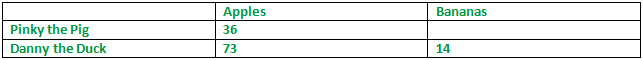The K5 Learning Blog urges parents to be pro-active in helping their children reach their full academic potential.

K5 Learning
provides an online reading and math program for kindergarten to grade 5 students.# How to Ace Math Problem Solving

When your kids struggle with their math, it’s time to take a step back and take a deep breath. They need to slow down and take their time. Here’s a step by step guide that will help your kids get through those tough math problems.

We’ll use a grade 3 addition word problem as an example to clarify:

Pinky the Pig bought 36 apples while Danny the Duck bought 73 apples and 14 bananas. How many apples do they have altogether?

Carefully read through the problem to make sure you understand what is being asked.

Pinky the pig and Danny the duck bought apples and bananas. The question is how many apples they have together.

Read through the problem again and as you read through it, make notes.

Pinky the pig –36 apples.
Danny the duck –73 apples and 14 bananas.
How many apples together?

## What is the problem asking

In your own words, say or write down exactly what the question is asking you to solve.

The question is asking how many apples the pig and the duck bought together.

## Write it down in detail

Go through the problem and write out the information in an organized fashion. A diagram or table might help.

##Turn it into math

Figure out what math operation(s) or formula(s) you need to use in order to solve this problem.

The problem wants us to add the number of apples Pinky the Pig and Danny the Duck have together. That means we need to make use of addition to add the apples.

## Find an example

Are you still struggling? Sometimes it’s hard to work out the solution, especially if the math problem involves several steps. It’s time to present the problem in an easier way. As teachers and parents we can often help our kids simplify the problem from our own math knowledge. If the problem is a bit harder, there are lots of resources online that you can look up for similar problems that have been worked out on paper or a video tutorial to watch.

In our example, let’s say the double-digit numbers are intimidating our student, so we’re going to simplify the equation for the sake of helping our student understand the operation needed.

Let’s say Pinky the Pig bought 3 apples and Danny the Duck 7 apples and 1 banana. Now, how many apples have they bought together? With 3 apples and 7 apples bought, the total number of apples is 10.

## Work out the problem

Now that we have got to the bottom of what is being asked and know what operation to use, it’s time to work out the problem.

Pinky the Pig bought 36 apples.
Danny the Duck bought 73 apples. (The 14 bananas do not matter)
We need to add up the apples.
36 + 73 = 109

Let’s round the numbers: 30 + 70 = 100. That is close to the exact number so it’s in the correct range.

The beauty of the basic operations is that addition and subtraction can be used to check answers too.

If we use the sum and take away one of the numbers, it should equal the other number.

109 – 73 = 36
109 – 36 = 73

If our student did not work out the sum correctly, we would not come to these sums.

(By the way, the same can be done with multiplication and division.)

Finally, go back and review the problem one last time. By going over the concepts, operations and formulas, it will help your kids to internalize the process and help them tackle harder math problems in the future.